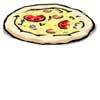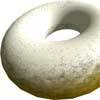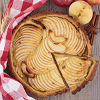#### You may also like### Pizza Portions

My friends and I love pizza. Can you help us share these pizzas equally?### Doughnut

How can you cut a doughnut into 8 equal pieces with only three cuts of a knife?### Pies

Grandma found her pie balanced on the scale with two weights and a quarter of a pie. So how heavy was each pie?

# Orange Drink

##### Age 7 to 11Challenge Level

You might have had to read this problem over several times before you understood it clearly - it wasn't easy! However, we have received many correct solutions and the majority of you tackled it in the same way. Esther explains how she went about solving the problem very well:

First I found out how much juice there is in each cup of drink. The 750mls in the bottle makes 15 cups so each cup contains 750/15=50mls.
This means that each cup contains 250-50=200mls of water.
10 litres=10,000mls. We need to know how many cups this is. As each cup holds 250mls we need 10,000/250=40 cups.
Each cup holds 200mls of water so 40 cups will need 200 times 40=8,000mls or 8 litres of water.

Esther also wrote:

There is another way using fractions.
The fraction of water in each cup is 200/250 or 4/5. This means that only 4/5 of the 10 litres is water. 1/5 of 10 is 2, so 4/5 is 8, and the answer is 8 litres.

Excellent reasoning Esther, thank you. Pupils from Queens College Junior School used a similar method to the first way Esther described. Greg, Liam, George and Joe from Moorfield Junior School found a slightly different way:

First we found out how much orange squash was in each 250 ml glass. We did this by sharing the 750 ml into the fifteen glasses. This gave us 50 ml in each glass. We then knew that there must be 200 ml water in each glass (250-50).

We then worked out how much water was in one litre of drink. This was 800 ml (200ml times 4).
Finally to find out how much water was in 10 litres we multiplied 800 ml by 10.
So the solution is that in 10 litres of drink there is 8000 ml (8 litres) of water. So we also worked out that there must be 2 litres of squash in the drink.

Alex from Columbia Independent School, MO, USA worked at the problem in a similar way to Esther's second method:

Fifteen glasses contain 3750 ml because each glass has 250 ml and 250 * 15 = 3750 ml.

Since we have only 750 ml of Orange drink, then we have 3000 ml, or 3 litres, of water.
So then each glass contains 200 ml of water which means that 80% of the diluted drink is water, because 200 is 80% of 250.
Then I found out that 4 glasses are equal to 1000 ml then I knew that 200 * 4 = 800. I knew that to prepare 1000 ml of orange drink I need 800 ml of water.
So 10 litres of orange drink need 8000 ml, or 8 litres, of water.

Finally, pupils from Wilby School tackled the problem in a slightly different way again. Here is Georgina's solution:

If you have a 750ml bottle of concentrated orange juice you can make 15 cups of juice. Each cup contains 250ml of juice.
I am trying to make 40 cups of juice, I need to know how much water I need.
If I make 15 cups of juice I need 750ml of juice and 3000ml of water.
If Imake 30 cups of water Ineed 1500ml of juice and 6000ml of water.
I cannot make 40 by adding 15 and 30 together so Ihave to make 10 cups. I only need to find out how much water Ineed to make 10 cups because if Iadd how much water Ihave in 30 cups and how much water Ihave in 10 cups together I will get 40 cups worth of water.
In 10 cups there is 2000ml of water. 6000ml + 2000ml = 8000ml.
There are 8000ml of water in 40 cups of juice.

Thank you very much to all those who sent solutions - even if there isn't room here to mention you personally.